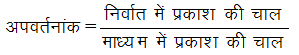Ex﻿

# 4. Light Refraction

#### Refractive Index

Refractive Index
(a) Refractive Index in terms of Speed of Light:
The refractive index of a medium may be defined in terms of the speed of light as follows :OR(b) Refractive Index in terms of Wavelength :
Since the frequency () remains unchanged when light passes from one medium to another, therefore,(c) Relative Refractive Index :
The relative refractive index of medium 2 with respect to medium 1 is defined as the ratio of speed of light (V1) in the medium 1 to the speed of light (V2) in medium 2 and is denoted by 1µ2.-
Thus,As refractive index is the ratio of two similar physical quantities, so it has no unit and dimension. Factors On Which The Refractive Index Of A Medium Depends Are :
(i) Nature of the medium.
(ii) Wavelength of the light used.
(iii) Temperature
(iv) Nature of the surrounding medium.
It may be noted that refractive index is a characteristic of the pair of the media and also depends on the wavelength of light, but is independent of the angle of incidence.

If you want to give information about online courses to other students, then share it with more and more on Facebook, Twitter, Google Plus. The more the shares will be, the more students will benefit. The share buttons are given below for your convenience.
×

#### NTSE Physics (Class X)

• Electricity
• Magnetic Effect of Curent
• Light Reflection
• Light Refraction
• Human Eye & Colourful World
• Source of Energy
• Motion

#### NTSE Physics (Class IX)

• Force & laws of motion
• Work and Energy
• Gravitation
• Sound

SHOW CHAPTERS